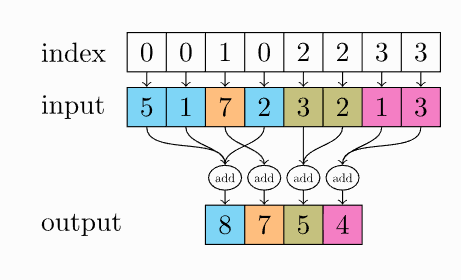# Torch index cumulative sum operation

Is there any way to create the torch.index_add behavior but for a cumulative summation?

For example instead of the final vector output in the following aggregation:I would like a cumulative index sum output vector as:

output: [5, 5+1, 7 ,5+1+2 , 3, 3+2, 1, 1+3]

Thank you!